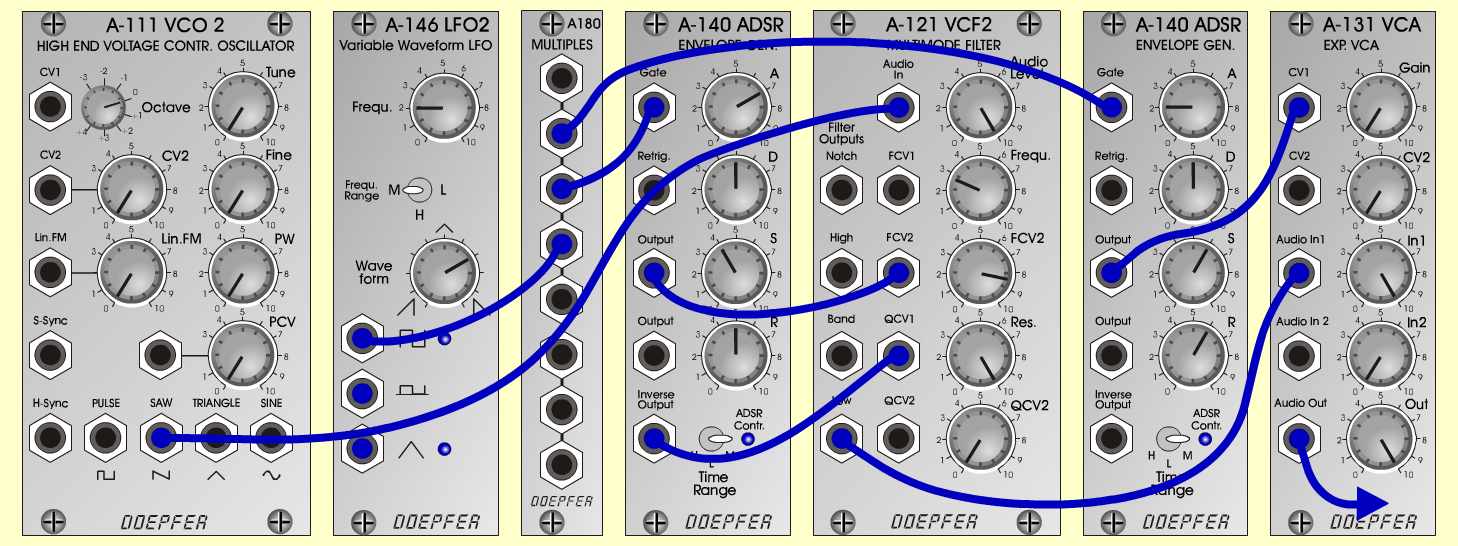DreamBrassInstrumentsD o e p f e r A - 100 Connections: Settings: DreamBrass A-111 (Saw)  <=>  A-121 (Audio In)   A-121 (Low)  <=>  A-131 (Audio In)   A-140/1 (Output)  <=>  A-121 (FCV 2)   A-140/1 (Inverse Output)  <=>  A-121 (QCV 1)   A-140/2 (Output)  <=>  A-131 (CV 1)   Trigger and Gate: KB, Seq or   A-146 (Square)  <=>  A-180   A-180  <=>  A-140/1 (Gate)   A-180  <=>  A-140/2 (Gate)   Pitch-Voltage: KB or Seq A-121 (Audio Level = 10, Freq = 2.8,   FCV 2 = 8.4, Res = 10)   A-140/1 (A = 7, D = 5, S = 4, R = 5, Range = M)   A-140/2 (A = 2, D = 5, S = 6, R = 6, Range = M)   A-131 (Gain = 0, Audio In 1 = 10,  Audio Out = 10)   A-146 (Freq = 2, Wave Form = 7, Range = M) DreamBrass 2 A-121 (Audio Level = 10, Freq = 5,   FCV 2 = 7, Res = 10)   A-140/1 (A = 1, D = 3, S = 5, R = 5, Range = M)   A-140/2 (A = 2, D = 5, S = 6, R = 6, Range = M)   A-131 (Gain = 0, Audio In 1 = 10,  Audio Out = 10)   A-146 (Freq = 2, Wave Form = 1, Range = M) DreamBrass 3 A-121 (Audio Level = 10, Freq = 3.0,   FCV 2 = 8, Res = 10)   A-140/1 (A = 1.5, D = 5, S = 5, R = 5, Range = M)   A-140/2 (A = 2, D = 5, S = 6, R = 6, Range = M)   A-131 (Gain = 0, Audio In 1 = 10,  Audio Out = 10)   A-146 (Freq = 2, Wave Form = 3, Range = M) Josef MuellerSound samples DreamBrass (130 Hz) DreamBrass (262 Hz) DreamBrass (523 Hz) DreamBrass 2 (130 Hz) DreamBrass 2 (262 Hz) DreamBrass 2 (523 Hz) DreamBrass 3 (130 Hz) DreamBrass 3 (262 Hz) DreamBrass 3 (523 Hz)